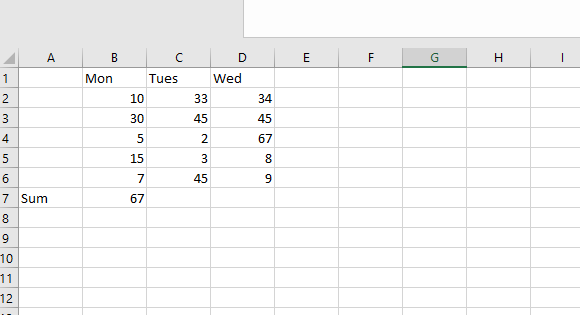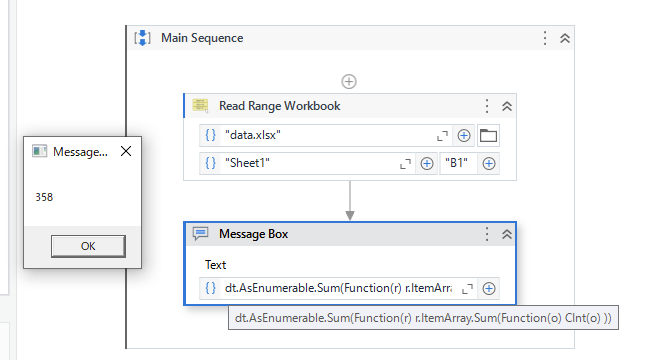# How to Automatic add the sum of columns values in a same row in UiPath Studio Coding?

How to Automatic add the sum of columns values in a same row in UiPath Studio Coding and also please activities should i use and drag & drop for this? like in Screen Shot i want Bot to calculate sum of all three columns B, C, & D. at onceGive a try to this.
CalculateSum.xaml (7.5 KB)

Hope this helps.

@raghav.bhardwaj.external

You can actually use excel formula for that and write the formula using write cell activity

for example for column B use write cell with cell value as `"B7"` and value to write as "`=Sum(B2:B6)"`

Similarly for other cells

cheers

Hi,`````` dt.AsEnumerable.Sum(Function(r) r.ItemArray.Sum(Function(o) CInt(o) ))